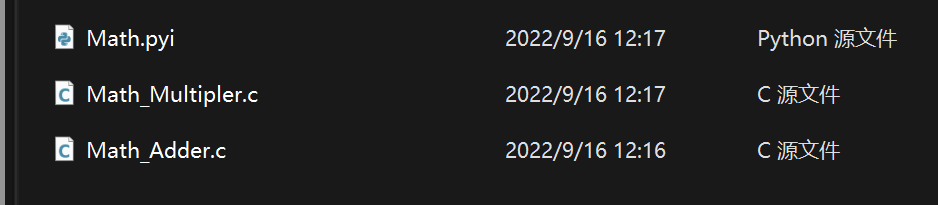# 7.2. PikaPython C 模块开发流程

## 7.2.2. 编写类接口

```# Math.pyi
def byInt(self, a:int, b:int)->int:
pass
def byFloat(self, a:float, b:float)->float:
pass
```

```# Math.pyi
def byInt(self, a:int, b:int)->int:...
def byFloat(self, a:float, b:float)->float:...
```

python 中类的方法的第一个参数都是 `self` 这是 python 的语法所要求的。

```# Math.pyi
class Multiplier:
def byInt(self, a:int, b:int)->int:
pass
def byFloat(self, a:float, b:float)->float:
pass
```

```# main.py
import Math
```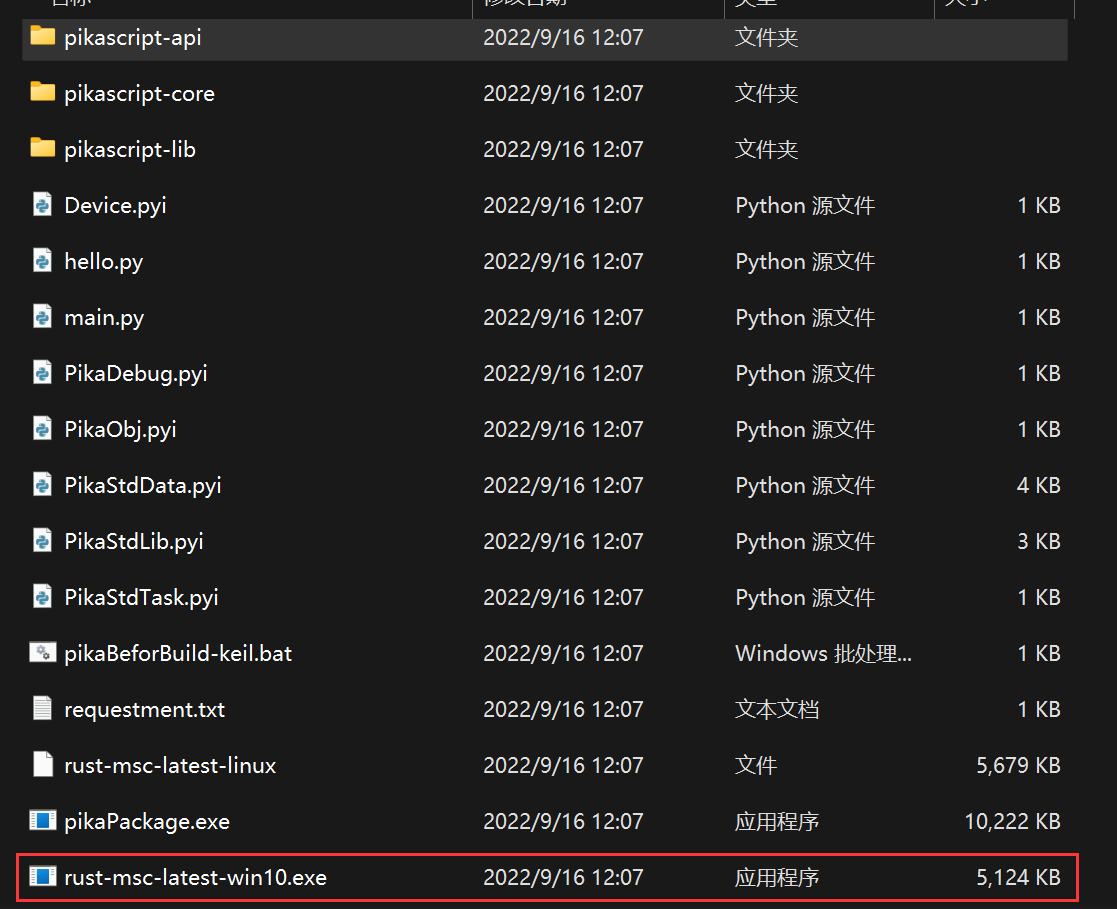## 7.2.3. 编写类的实现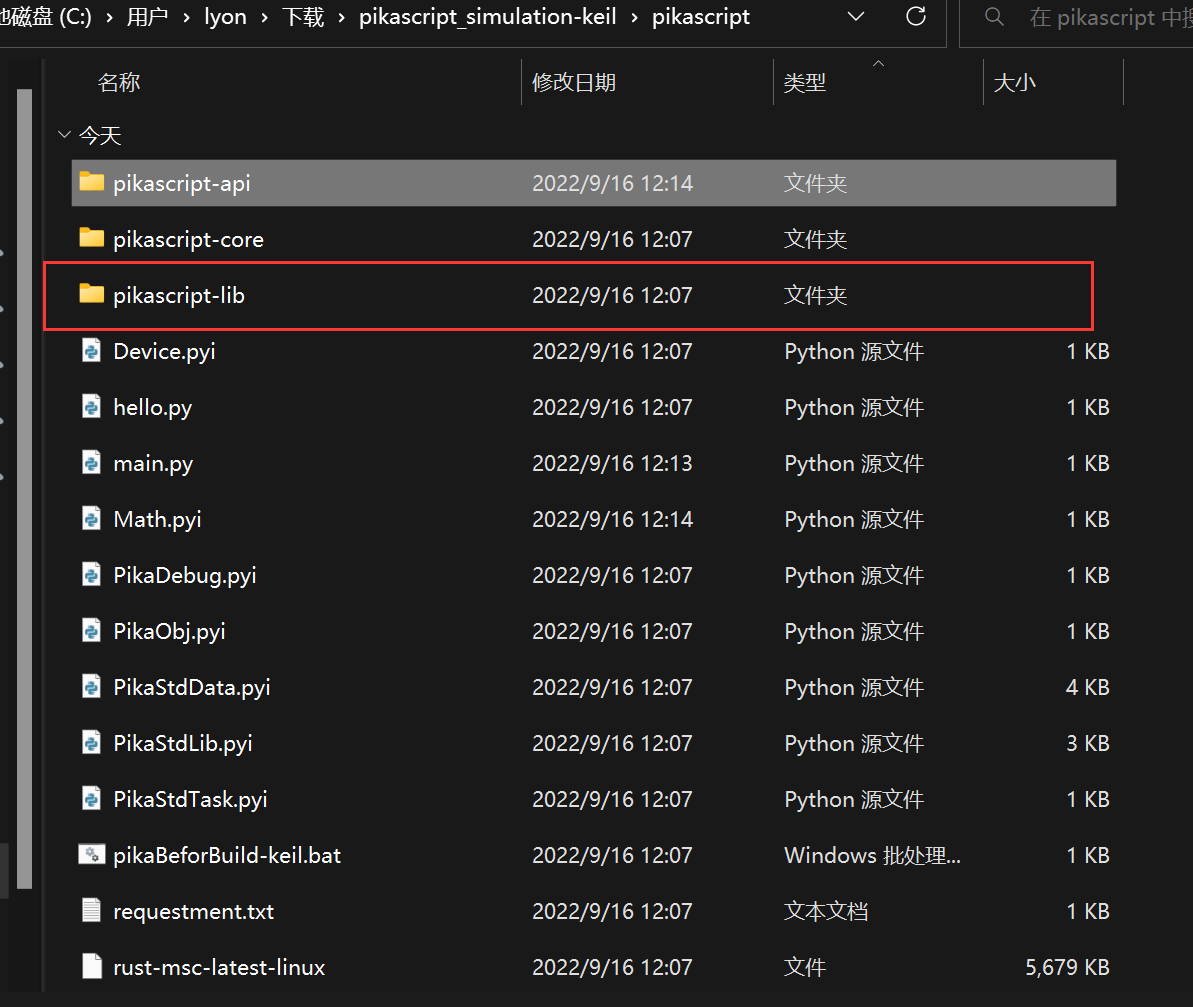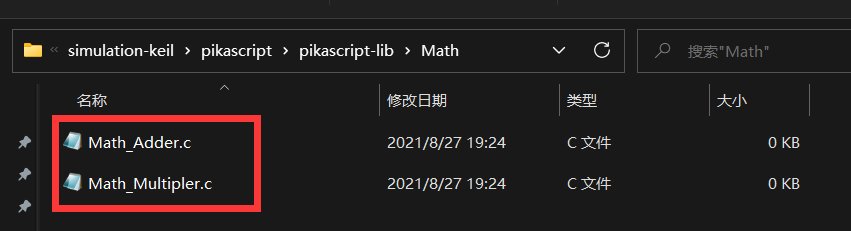```/* Math_Multiplier.h */
/* ******************************** */
/* Warning! Don't modify this file! */
/* ******************************** */
#ifndef __Math_Multiplier__H
#define __Math_Multiplier__H
#include <stdio.h>
#include <stdlib.h>
#include "PikaObj.h"

PikaObj *New_Math_Multiplier(Args *args);

double Math_Multiplier_byFloat(PikaObj *self, double a, double b);
int Math_Multiplier_byInt(PikaObj *self, int a, int b);

#endif
```
```/* Math_Adder.h */
/* ******************************** */
/* Warning! Don't modify this file! */
/* ******************************** */
#include <stdio.h>
#include <stdlib.h>
#include "PikaObj.h"

double Math_Adder_byFloat(PikaObj *self, double a, double b);
int Math_Adder_byInt(PikaObj *self, int a, int b);

#endif
```

```/* Math_Adder.c */
#include "pikaScript.h"

double Math_Adder_byFloat(PikaObj *self, double a, double b)
{
return a + b;
}

int Math_Adder_byInt(PikaObj *self, int a, int b)
{
return a + b;
}
```
```/* Math_Multipler.c */
#include "pikaScript.h"

double Math_Multiplier_byFloat(PikaObj *self, double a, double b)
{
return a * b;
}

int Math_Multiplier_byInt(PikaObj *self, int a, int b)
{
return a * b;
}
```

## 7.2.4. 测试一下效果

```# main.py
import Math

muler = Math.Multiplier()

print('1 + 2')
print(res1)

print('2.3 + 4.2')
print(res2)

res3 = muler.byInt(2, 3)
print('2 * 3')
print(res3)

res4 = muler.byFloat(2.3, 44.2)
print('2.3 * 44.2')
print(res4)
```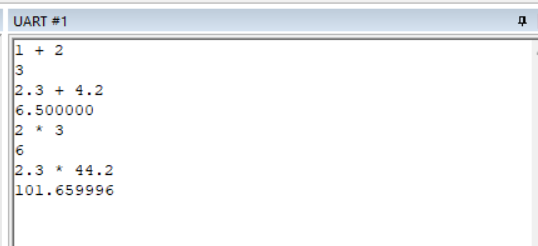## 7.2.5. 可用的类型注解

Python 类型注解 C 原生类型 说明
int int python 基本类型
int64 int64_t 64 位整型
float double python 基本类型
str char * python 基本类型
bytes uint8_t * python 基本类型
pointer void * PikaPython 特有的类型注解
any Arg* PikaPython 提供的泛型容器

1. `str` 作为返回值时，在 c 中返回的是 `char*` ，如果要返回的字串是函数中的局部变量，需要用 `obj_cacheStr` 进行缓存，避免出函数作用域后发生悬垂引用，如: `return obj_cacheStr(self, res);`

2. `bytes` 作为返回值时，在 c 中返回的是 `Arg*`，这是因为 `bytes` 需要指定长度，返回 `uint8_t*` 不能达到要求。正确返回方式为：`return arg_newBytes(bytes, len);`

## 7.2.6. 发布模块